xTerminal velocityOverview

In fluid dynamics
Fluid dynamics
In physics, fluid dynamics is a sub-discipline of fluid mechanics that deals with fluid flow—the natural science of fluids in motion. It has several subdisciplines itself, including aerodynamics and hydrodynamics...

an object is moving at its terminal velocity if its speed is constant due to the restraining force exerted by the fluid through which it is moving.

A free-falling object achieves its terminal velocity when the downward force of gravity (FG) equals the upward force of drag
Drag (physics)
In fluid dynamics, drag refers to forces which act on a solid object in the direction of the relative fluid flow velocity...

(Fd). This causes the net force
Net force
In physics, net force is the total force acting on an object. It is calculated by vector addition of all forces that are actually acting on that object. Net force has the same effect on the translational motion of the object as all actual forces taken together...

on the object to be zero, resulting in an acceleration of zero.

As the object accelerates (usually downwards due to gravity), the drag force acting on the object increases, causing the acceleration to decrease.Unanswered QuestionsDiscussionsEncyclopediaIn fluid dynamics
Fluid dynamics
In physics, fluid dynamics is a sub-discipline of fluid mechanics that deals with fluid flow—the natural science of fluids in motion. It has several subdisciplines itself, including aerodynamics and hydrodynamics...

an object is moving at its terminal velocity if its speed is constant due to the restraining force exerted by the fluid through which it is moving.

A free-falling object achieves its terminal velocity when the downward force of gravity (FG) equals the upward force of drag
Drag (physics)
In fluid dynamics, drag refers to forces which act on a solid object in the direction of the relative fluid flow velocity...

(Fd). This causes the net force
Net force
In physics, net force is the total force acting on an object. It is calculated by vector addition of all forces that are actually acting on that object. Net force has the same effect on the translational motion of the object as all actual forces taken together...

on the object to be zero, resulting in an acceleration of zero.

As the object accelerates (usually downwards due to gravity), the drag force acting on the object increases, causing the acceleration to decrease. At a particular speed, the drag force produced will equal the object's weight. At this point the object ceases to accelerate altogether and continues falling at a constant speed called terminal velocity (also called settling velocity). Terminal velocity varies directly with the ratio of weight to drag. More drag means a lower terminal velocity, while increased weight means a higher terminal velocity. An object moving downward with greater than terminal velocity (for example because it was affected by a downward force or it fell from a thinner part of the atmosphere or it changed shape) will slow until it reaches terminal velocity.

## Examples

Based on wind resistance, for example, the terminal velocity of a skydiver in a belly-to-earth (ie:face down) free-fall
Free-fall
Free fall is any motion of a body where gravity is the only force acting upon it, at least initially. These conditions produce an inertial trajectory so long as gravity remains the only force. Since this definition does not specify velocity, it also applies to objects initially moving upward...

position is about 195 km/h (122 mph
Miles per hour
Miles per hour is an imperial unit of speed expressing the number of statute miles covered in one hour. It is currently the standard unit used for speed limits, and to express speeds generally, on roads in the United Kingdom and the United States. It is also often used to express the speed of...

or 54 m/s). This velocity is the asymptotic
Asymptote
In analytic geometry, an asymptote of a curve is a line such that the distance between the curve and the line approaches zero as they tend to infinity. Some sources include the requirement that the curve may not cross the line infinitely often, but this is unusual for modern authors...

limiting value of the acceleration process, because the effective forces on the body balance each other more and more closely as the terminal velocity is approached. In this example, a speed of 50% of terminal velocity is reached after only about 3 seconds, while it takes 8 seconds to reach 90%, 15 seconds to reach 99% and so on.

Higher speeds can be attained if the skydiver pulls in his or her limbs (see also freeflying
Freeflying
Freeflying is a skydiving discipline which began in 1990 when Olav Zipser began experimenting with non-traditional forms of body flight. Zipser formed the Freefly Clowns as a two person competitive team with Mike Vail in 1992, and was joined by Omar Alhegelan, Charles Bryan, and Stefania...

). In this case, the terminal velocity increases to about 320 km/h (200 mph or 90 m/s), which is almost the terminal velocity of the Peregrine Falcon
Peregrine Falcon
The Peregrine Falcon , also known as the Peregrine, and historically as the Duck Hawk in North America, is a widespread bird of prey in the family Falconidae. A large, crow-sized falcon, it has a blue-gray back, barred white underparts, and a black head and "moustache"...

diving down on its prey. The same terminal velocity is reached for a typical .30-06
.30-06 Springfield
The .30-06 Springfield cartridge or 7.62×63mm in metric notation, was introduced to the United States Army in 1906 and standardized, and was in use until the 1960s and early 1970s. It replaced the .30-03, 6 mm Lee Navy, and .30 US Army...

bullet dropping downwards—when it is returning to earth having been fired upwards, or dropped from a tower—according to a 1920 U.S. Army Ordnance study.

Competition speed skydivers fly in the head down position and reach even higher speeds. The current world record is 614 mph (988 km/h) by Joseph Kittinger
Joseph Kittinger
Joseph William Kittinger II is a former Command Pilot and career military officer in the United States Air Force. He is most famous for his participation in Project Manhigh and Project Excelsior, holding the records for having the highest, fastest and longest skydive, from a height greater than...

, set at high altitude where the lesser density of the atmosphere decreased drag.

An object falling toward the surface of the Earth will fall 9.81 meters (or 32.18 feet) per second faster every second (an acceleration of 9.81 m/s² or 32.18 ft/s²). The reason an object reaches a terminal velocity is that the drag force resisting motion is approximately proportional to the square of its speed. At low speeds, the drag is much less than the gravitational force and so the object accelerates. As it accelerates, the drag increases, until it equals the weight. Drag also depends on the projected area. This is why objects with a large projected area relative to mass, such as parachutes, have a lower terminal velocity than objects with a small projected area relative to mass, such as bullets.

Mathematically, terminal velocity—without considering buoyancy
Buoyancy
In physics, buoyancy is a force exerted by a fluid that opposes an object's weight. In a column of fluid, pressure increases with depth as a result of the weight of the overlying fluid. Thus a column of fluid, or an object submerged in the fluid, experiences greater pressure at the bottom of the...

effects—is given by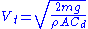where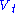= terminal velocity,= mass of the falling object,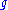= acceleration due to gravity
Earth's gravity
The gravity of Earth, denoted g, refers to the acceleration that the Earth imparts to objects on or near its surface. In SI units this acceleration is measured in metres per second per second or equivalently in newtons per kilogram...

,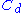= drag coefficient
Drag coefficient
In fluid dynamics, the drag coefficient is a dimensionless quantity that is used to quantify the drag or resistance of an object in a fluid environment such as air or water. It is used in the drag equation, where a lower drag coefficient indicates the object will have less aerodynamic or...

,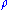= density
Density
The mass density or density of a material is defined as its mass per unit volume. The symbol most often used for density is ρ . In some cases , density is also defined as its weight per unit volume; although, this quantity is more properly called specific weight...

of the fluid through which the object is falling, and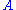= projected area of the object.

Mathematically, an object approaches its terminal velocity asymptotically
Asymptote
In analytic geometry, an asymptote of a curve is a line such that the distance between the curve and the line approaches zero as they tend to infinity. Some sources include the requirement that the curve may not cross the line infinitely often, but this is unusual for modern authors...

.

Buoyancy effects, due to the upward force on the object by the surrounding fluid, can be taken into account using Archimedes' principle
Buoyancy
In physics, buoyancy is a force exerted by a fluid that opposes an object's weight. In a column of fluid, pressure increases with depth as a result of the weight of the overlying fluid. Thus a column of fluid, or an object submerged in the fluid, experiences greater pressure at the bottom of the...

: the masshas to be reduced by the displaced fluid mass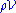, with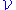the volume of the object. So instead ofuse the reduced mass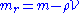in this and subsequent formulas.

On Earth, the terminal velocity of an object changes due to the properties of the fluid, the mass of the object and its projected cross-sectional surface area.

Air density increases with decreasing altitude, ca. 1% per 80 metres (262 ft) (see barometric formula
Barometric formula
The barometric formula, sometimes called the exponential atmosphere or isothermal atmosphere, is a formula used to model how the pressure of the air changes with altitude.-Pressure equations:...

). For objects falling through the atmosphere, for every 160 metres (525 ft) of falling, the terminal velocity decreases 1%. After reaching the local terminal velocity, while continuing the fall, speed decreases to change with the local terminal velocity.

### Derivation for terminal velocity

Mathematically, defining down to be positive, the net force acting on an object falling near the surface of Earth is (according to the drag equation
Drag equation
In fluid dynamics, the drag equation is a practical formula used to calculate the force of drag experienced by an object due to movement through a fully enclosing fluid....

):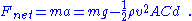At equilibrium, the net force is zero (F = 0);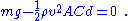Solving for v yields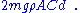## Terminal velocity in the presence of buoyancy force

When the buoyancy effects are taken into account, an object falling through a fluid under its own weight can reach a terminal velocity (settling velocity) if the net force acting on the object becomes zero. When the terminal velocity is reached the weight of the object is exactly balanced by the upward buoyancy force and drag force. That is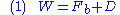where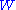= weight of the object,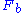= buoyancy force acting on the object, and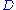= drag force acting on the object.

If the falling object is spherical in shape, the expression for the three forces are given below: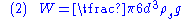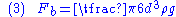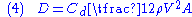where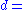diameter of the spherical object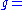gravitational acceleration,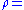density of the fluid,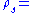density of the object,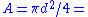projected area of the sphere,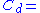drag coefficient, and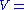characteristic velocity (taken as terminal velocity,).

Substitution of equations (2–4) in equation (1) and solving for terminal velocity,to yield the following expression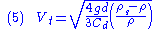.

### Terminal velocity in creeping flow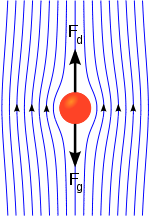For very slow motion of the fluid, the inertia forces of the fluid are negligible (assumption of massless fluid) in comparison to other forces. Such flows are called creeping flows and the condition to be satisfied for the flows to be creeping flows is the Reynolds number,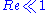. The equation of motion for creeping flow (simplified Navier-Stokes equation) is given by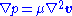where= velocity vector field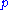= pressure field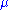= fluid viscosity
Viscosity
Viscosity is a measure of the resistance of a fluid which is being deformed by either shear or tensile stress. In everyday terms , viscosity is "thickness" or "internal friction". Thus, water is "thin", having a lower viscosity, while honey is "thick", having a higher viscosity...

The analytical solution for the creeping flow around a sphere was first given by Stokes
George Gabriel Stokes
Sir George Gabriel Stokes, 1st Baronet FRS , was an Irish mathematician and physicist, who at Cambridge made important contributions to fluid dynamics , optics, and mathematical physics...

in 1851. From Stokes' solution, the drag force acting on the sphere can be obtained as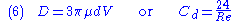where the Reynolds number,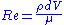. The expression for the drag force given by equation (6) is called Stokes' law
Stokes' law
In 1851, George Gabriel Stokes derived an expression, now known as Stokes' law, for the frictional force – also called drag force – exerted on spherical objects with very small Reynolds numbers in a continuous viscous fluid...

.

When the value ofis substituted in the equation (5), we obtain the expression for terminal velocity of a spherical object moving under creeping flow conditions: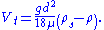### Applications

The creeping flow results can be applied in order to study the settling of sediment particles near the ocean bottom and the fall of moisture drops in the atmosphere. The principle is also applied in the falling sphere viscometer, an experimental device used to measure the viscosity of high viscous fluids.

## External links

The source of this article is wikipedia, the free encyclopedia.  The text of this article is licensed under the GFDL.